(1)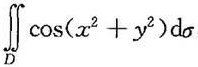，其中D为圆环形域π/3≤x2+y2≤π;

(2)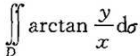，其中D为由不等式1≤x2+y2≤4、y≥0及y≤x所决定的区域;

(3)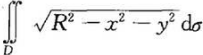，其中D为圆域x2+y2≤Rx;

(4)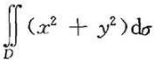，其中D为由双纽线(x2+y2)2=a2(x2-y2)所围成的封闭区域。

• 设< A,＋,‧>是一个环,并且对于任意的a∈A.都有a‧a=a,证明: a)对于任意的a∈A.都有a＋a=θ,其中θ是加法幺元。 b)< A,＋,‧>是可交换环。

• 设f(1,2)=4,d(1,2)=16dx+4dy,d(1,4)=64dx+8dy.令z=f[x,(x,y)],求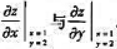.

• 证明:xd<／sup>－1整除xn<／sup>－1必要且只要d整除n。

• 经济全球化的核心是资源配置的全球化和市场的()

A.一体化

B.整合化

C.多元化

D.综合化

•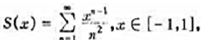计算积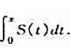• 秘书参谋活动的内涵,主要指向领导者的()

A.全部职能

B.管理职能

C.基本职能

D.指挥职能